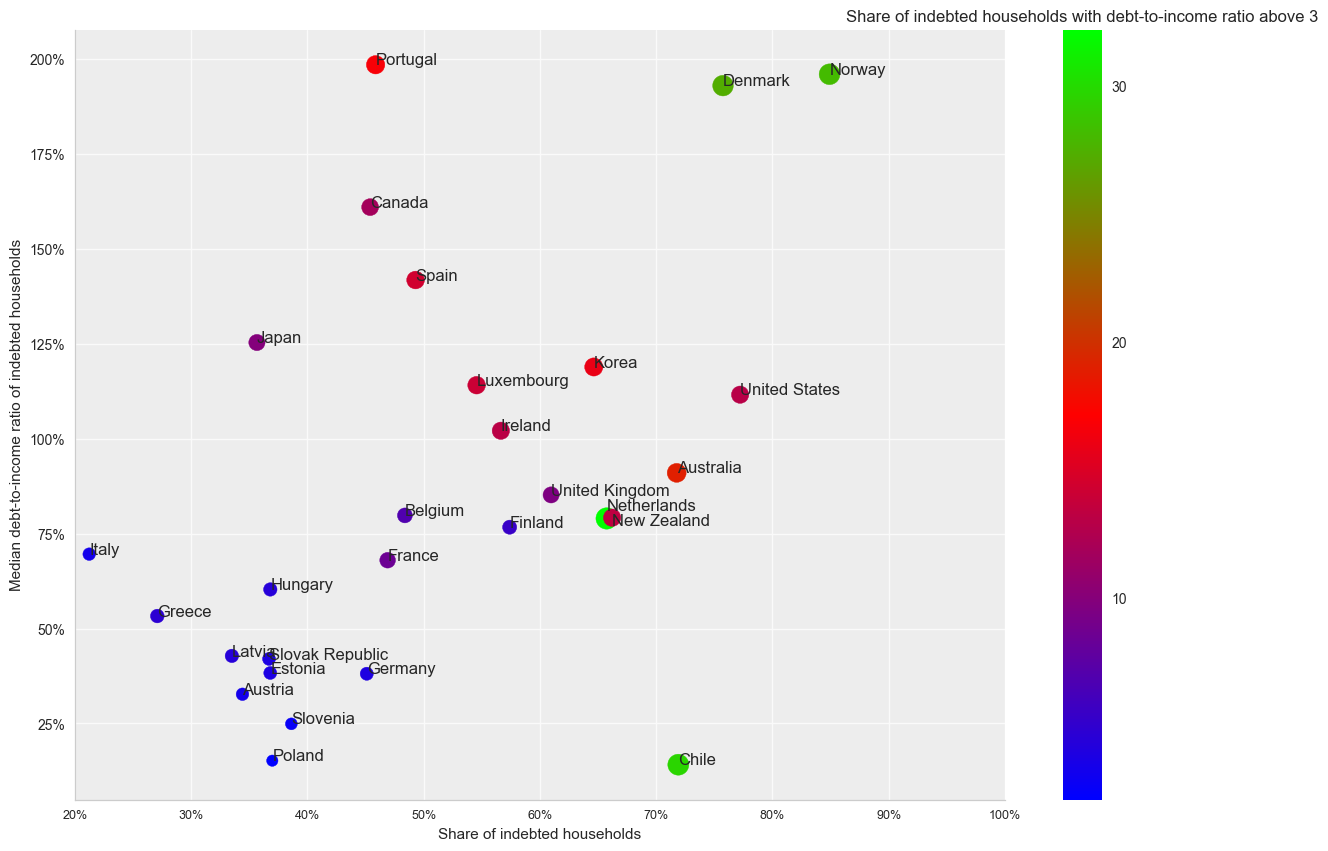# Wealth Across OECD countries¶

The OECD recently published updated statistics for wealth distribution across OECD countries. Below are some facts that show how wealth is distributed, how much share of total wealth do the richest households own, how many households are in debt, and how many individuals are asset poor.

Facts about wealth distribution accross OECD countries

*The average household wealth in 2010 PPP terms is 258,600 USD

*The richest OECD countries across are the US, UK and Luxemburg

*The poorest OECD countries are Latvia, Chile, and Hungary

*The richest 1% of households owe, on average, 52% of total wealth

*In the US, the richest 1% of households owe, approximately,79% of total wealth

*Overall, most household wealth is non-financial and is derived from home ownership

The published dataset is available at:

http://stats.oecd.org/

The dataset is titled Wealth Distribution and can be found under Social Protection and Well Being.

In addition, we will need data on PPP and CPI, available at:

Note that for the CPI we will use the Consumer prices - all items and for PPP the Purchasing Power Parities for private consumption.

### Preface¶

The following notebook provides the code to replicate the descriptive statistics and graphs. I currently use the following libraries: matplotlib, numpy, pandas, and seaborn.

In :
# -*- coding: utf-8 -*-
"""
Created on Dec  8 15:47:44 2017
"""

#Import libs
import numpy as np

import pandas as pd
from  pandas.plotting import scatter_matrix

import matplotlib.pyplot as plt
import matplotlib.pyplot as plt; plt.rcdefaults()
import matplotlib.ticker as mtick

import seaborn as sns


### Functions¶

I define a couple of functions which I have used in the past to filter data and edit plots. These are used later in the code.

In :
def filter_data(df, variable=None, country=None, code=None, year=None):
"""""
The following function filters the data frame. It is able to filter it by several parameters.
This is useful as the data is in long form and I need to filter it and reshape.
"""""
if variable is not None:
df=df.loc[df['Variable']==variable,:]
if code is not None:
df=df.loc[df['VAR']==code,:]

if country is not None:
df=df.loc[df['COUNTRY']==country,:]

if year is not None:
df=df.loc[df['time']==year,:]

obs=len(df)
print ('The filtering return %d obs, for var %s, country %s, and year %s,code %s'
% (obs,variable ,country,year, code))
return df

def remove_ticks(ax):
ax.xaxis.set_ticks_position('none')
ax.yaxis.set_ticks_position('none')

def remove_splines(ax, spl):
for s in spl:
ax.spines[s].set_visible(False)

def modify_splines(ax, lwd, col):
for s in ['bottom', 'left','top','right']:
ax.spines[s].set_linewidth(lwd)
ax.spines[s].set_color(col)

def change():
"""""
Modifies the plot to visual standard used in other project
"""""
remove_ticks(ax)
modify_splines(ax, lwd=0.9, col='0.8')
remove_splines(ax, ['top','right'])
ax.patch.set_facecolor('0.93')
ax.grid(True, 'major', color='0.98', linestyle='-', linewidth=1.0)
ax.set_axisbelow(True)


# Config¶

In :
#Setting the directory

#There are 3 files that we need. See source above for details.

#Note we only need a subsection of the ppp and cpi data. We keep the specific data and columns
columns=['LOCATION','TIME','Value']

ppp=ppp.loc[ppp['Transaction']=="Purchasing Power Parities for private consumption",columns]
cpi=cpi.loc[cpi['Subject']=="Consumer prices - all items",:]
cpi=cpi.loc[cpi['Measure']=="Index",columns]

#Rename columns as they have the same name
ppp=ppp.rename(columns={'Value':'ppp'})
cpi=cpi.rename(columns={'Value':'cpi'})

#We merge the files with the original data set
data=wealth.merge(ppp, how='left',left_on=['COUNTRY', 'Time'], right_on=['LOCATION','TIME'])
df=data.merge(cpi, how='left',left_on=['COUNTRY', 'Time'], right_on=['LOCATION','TIME'])


### Data Transformation¶

Note that the OECD data is presented in current values in local currency. In order to do a proper comparison between countries and across time, we need to deflate it and define it in ppp terms.

In :
#List all variables available
df.Variable.unique()

Out:
array(['Mean financial assets per household (current prices)',
'Mean liabilities per household (current prices)',
'Mean net wealth per household (current prices)',
'Mean net wealth per person (current prices)',
'Mean non-financial assets per household (current prices)',
'Mean to median net wealth ratio',
'Median debt-to-assets ratio of indebted households',
'Median debt-to-income ratio of indebted households',
'Median net wealth per household (current prices)',
'Share of indebted households with debt-to-assets ratio above 75%',
'Share of bottom 60% of wealth', 'Share of indebted households',
'Share of indebted households with debt-to-income ratio above 3',
'Share of individuals with eq. liquid financial wealth <25% of income poverty line',
'Share of individuals with eq. liquid financial wealth <50 % of  income poverty line',
'Share of individuals with eq. net wealth < 25% of income poverty line',
'Share of individuals with eq. net wealth <50 % of  income poverty line',
'Share of top 1% of wealth', 'Share of top 10% of wealth',
'Share of top 5% of wealth'], dtype=object)
In :
#Get the code for each variable
df.groupby(['Variable','VAR']).sum().reset_index()[['Variable','VAR']]

Out:
Variable VAR
0 Mean financial assets per household (current p... T3AC2
1 Mean liabilities per household (current prices) T3AC3
2 Mean net wealth per household (current prices) T1C5
3 Mean net wealth per person (current prices) MNWI
4 Mean non-financial assets per household (curre... T3AC1
5 Mean to median net wealth ratio M2MR
6 Median debt-to-assets ratio of indebted househ... T4C5
7 Median debt-to-income ratio of indebted househ... T4C4
8 Median net wealth per household (current prices) T1C7
9 Share of bottom 60% of wealth SB60
10 Share of indebted households PIH
11 Share of indebted households with debt-to-asse... PIH75
12 Share of indebted households with debt-to-inco... PIHR3
13 Share of individuals with eq. liquid financial... T6C2
14 Share of individuals with eq. liquid financial... T6C3
15 Share of individuals with eq. net wealth < 25%... T6C6
16 Share of individuals with eq. net wealth <50 %... T6C7
17 Share of top 1% of wealth ST1
18 Share of top 10% of wealth ST10
19 Share of top 5% of wealth ST5

### Transforming data into current ppp terms¶

In :
vars_to_mofify=[
'Mean financial assets per household (current prices)',
'Mean liabilities per household (current prices)',
'Mean net wealth per household (current prices)',
'Mean net wealth per person (current prices)',
'Mean non-financial assets per household (current prices)',
'Median net wealth per household (current prices)']

#Create ppp_current_values
df['tranformed_value']=df['Value']

#Transform them if they are in list.
for mod in vars_to_mofify:
print(mod)
df.loc[df['Variable']==mod, 'tranformed_value']=df['Value']/(df['cpi']/100)/df['ppp']

Mean financial assets per household (current prices)
Mean liabilities per household (current prices)
Mean net wealth per household (current prices)
Mean net wealth per person (current prices)
Mean non-financial assets per household (current prices)
Median net wealth per household (current prices)


# Data Description¶

Following the transforlation, we can proceed to describe the data

## Average Household Wealth¶

In :
#Filter the data from the master dataset. See code above for function and variable code.
mean_wealth=filter_data(df, code='T1C5')
median_wealth=filter_data(df, code='T1C7')

#Keeping only the latest observation. This sorts by year and keeps the most recent one
s_mean=mean_wealth.sort_values(['Country', 'Time'], ascending=False)

s_median=median_wealth.sort_values(['Country', 'Time'], ascending=False)

The filtering return 48 obs, for var None, country None, and year None,code T1C5
The filtering return 48 obs, for var None, country None, and year None,code T1C7

In :
#Average wealth across OECD countries
print('Mean Wealth',s_mean.tranformed_value.mean())
s_mean=s_mean.rename(columns={'tranformed_value':'mean'})

#Median wealth across OECD countries
print('Median Wealth',s_median.tranformed_value.mean())
s_median=s_median.rename(columns={'tranformed_value':'median'})

Mean Wealth 258626.52808147462
Median Wealth 123609.01117650872


### Graph 1- Household wealth¶

In :
#Join median and mean to graph them
obj=s_mean.merge(s_median, on=['Country','Time'], copy=False)[['Country','Time','mean','median']]
obj=obj.sort_values(by=['mean'])
y_pos = np.arange(len(obj))

plt.clf()
fig=plt.figure( figsize=(15, 5))

#Add bar chart of mean
bar=plt.bar(y_pos, obj['mean'],  alpha=.8, color='navy',  zorder=1, label='Mean')

#Add the mean of OECD
line_mean=plt.axhline(y=230000,  color='black',  zorder=1, linestyle='-' )
ax.annotate('OECD 28 Mean', xy=(2, 10000), xytext=(3, 258600),  )

scat=plt.scatter(y_pos, obj['median'],  marker='d', color='orange', alpha=1,  zorder=2, label='Median')

########### Graph Options
plt.xticks(y_pos, obj['Country'])
plt.xticks(rotation=60,  fontsize = 11, ha="right", fontname='Arial' )
plt.yticks( fontname='Arial', fontsize = 9 )
plt.ylabel('2010 PPP USD')
plt.title('')
plt.legend([bar,scat],['mean','median'])

#See function above
change()

plt.legend(bbox_to_anchor=(.2, 1.02, 0.6, .102), mode='expand',
ncol=2, borderaxespad=0, handles=[bar, scat])

plt.savefig(root+'f1.png')
plt.show()

<matplotlib.figure.Figure at 0xbcd55f8>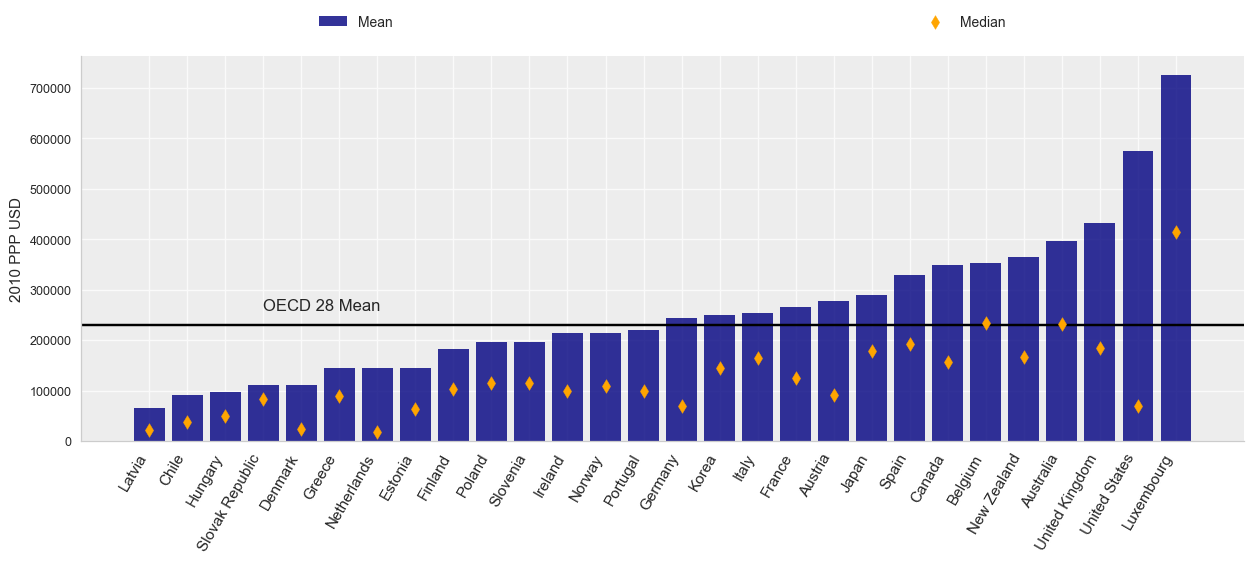## Composition of Wealth¶

In the following section, we analyze the components of household wealth. Specifically, how it is divided between financial and non-financial assets, and liabilities.

In :
#Filter Data
liabilities=filter_data(df, code='T3AC3')
f_assets=filter_data(df, code='T3AC2')
nf_assets=filter_data(df, code='T3AC1')

#Create a dictionary for transformation.
wealth_comp={'fin':f_assets,
'nofin':nf_assets,
'liab':liabilities,}

#Select latest year. Same as above but using a loop.
for f in wealth_comp:
print(f)
wealth_comp[f]=wealth_comp[f].sort_values(['Country', 'Time'], ascending=False)
wealth_comp[f]=wealth_comp[f].rename(columns={'tranformed_value':str(f)})

#Merge them together
comp=wealth_comp['fin'].merge(wealth_comp['nofin'], on=['Country','Time'], copy=False)[['Country','Time','fin','nofin']]
comp=comp.merge(wealth_comp['liab'], on=['Country','Time'], copy=False)[['Country','Time','fin','nofin','liab']]

#Calculate ownership
comp['liab']=comp['liab']*-1
comp['total']=comp['fin']+comp['nofin']+comp['liab']

#Express them as percentqges
comp['p_nf']=comp['nofin']/comp['total']
comp['p_f']=comp['fin']/comp['total']
comp['p_l']=comp['liab']/comp['total']
comp['unit']=comp['p_nf']+comp['p_f']+comp['p_l']

The filtering return 48 obs, for var None, country None, and year None,code T3AC3
The filtering return 48 obs, for var None, country None, and year None,code T3AC2
The filtering return 47 obs, for var None, country None, and year None,code T3AC1
fin
nofin
liab

Out:
Country Time fin nofin liab total p_nf p_f p_l unit
0 United States 2016 394396.140533 274923.455713 -93488.511543 575831.084703 0.477438 0.684916 -0.162354 1.0
1 United Kingdom 2015 213914.214778 269219.413765 -50808.841345 432324.787198 0.622725 0.494800 -0.117525 1.0
2 Spain 2012 84442.015816 286821.428538 -41957.397441 329306.046913 0.870987 0.256424 -0.127412 1.0
3 Slovenia 2014 57800.062542 148192.771601 -9416.382985 196576.451157 0.753868 0.294034 -0.047902 1.0
4 Slovak Republic 2014 16458.454523 102952.672368 -9010.615247 110400.511645 0.932538 0.149080 -0.081618 1.0

### Graph 2- Composition of wealth¶

In :
# CREATE THE FIGURE 2

plt.clf()
fig=plt.figure( figsize=(10, 8 ))

#Sorting data by non-financials
comp=comp.sort_values(by=['p_nf'])
y_pos = np.arange(len(comp))

#Plotting bar graphs, one per each part of wealth
p1=plt.barh(y_pos,comp['p_nf'], linewidth=5, color='navy', label='Non-Financial Weatlh', zorder=2)
#Note, one needs to add and change the z index so the bars are visible
p2=plt.barh(y_pos,comp['p_f']+comp['p_nf'], linewidth=5, color='peru', label='Financial Weatlh', zorder=1)
p3=plt.barh(y_pos,comp['p_l'], linewidth=5, color='darkred', label='Liabilities', left=0)
plt.yticks(y_pos,comp['Country'], fontsize = 9, ha="right", fontname='Arial')
change()

plt.legend([p1,p2,p3],['Non-Financial Weatlh','Financial Weatlh', 'Liabilities'], ncol=3
, bbox_to_anchor=(0, 1.02, 1.05, .102))
plt.xlim([-.9,1.5])

plt.savefig(root+'f2.png')
plt.show()

<matplotlib.figure.Figure at 0xc1167f0>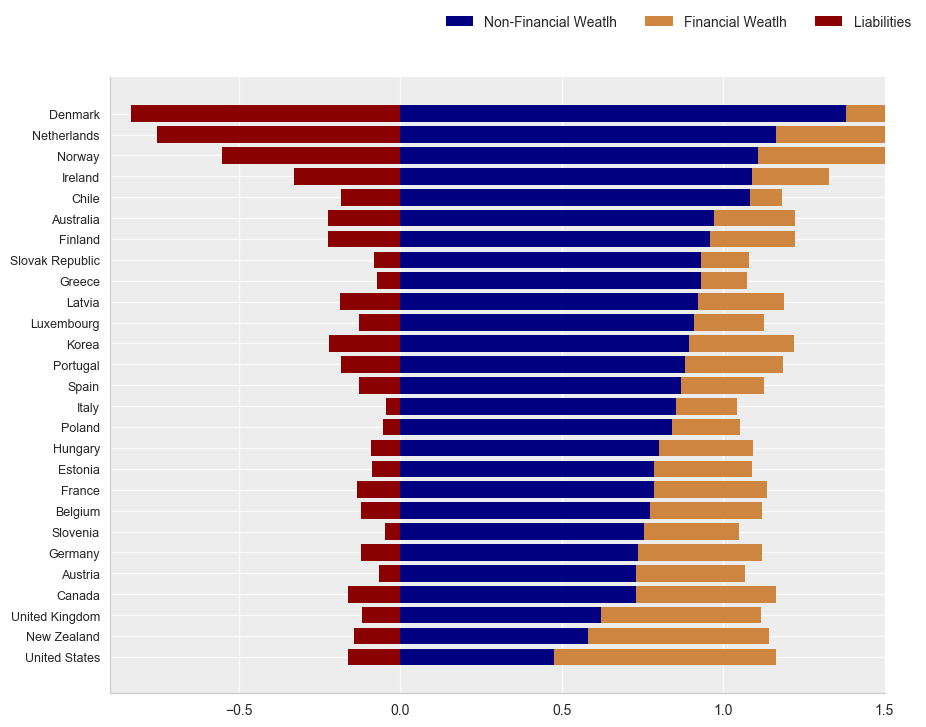### Mean over all OECD countries¶

In :
plt.clf()
fig=plt.figure( figsize=(5, 5 ))

x=comp[['p_nf','p_f','p_l']].mean().tolist()

colors=['navy','peru','darkred']

#For liabilities, change them to negative
x=x*-1

#Labels
lab=['Non-Financial Assets','Financial Assets','Liabilities']
explode = (0, 0, 0.1)
fig1, ax1 = plt.subplots()

patches, texts, autotexts = plt.pie(x,explode=explode, labels=None, autopct='%1.1f%%',
startangle=90, colors=colors,  )

ax1.axis('equal')  # Equal aspect ratio ensures that pie is drawn as a circle.

plt.savefig(root+'f3.png')
plt.show()

<matplotlib.figure.Figure at 0xc999e48>
<matplotlib.figure.Figure at 0xc999160>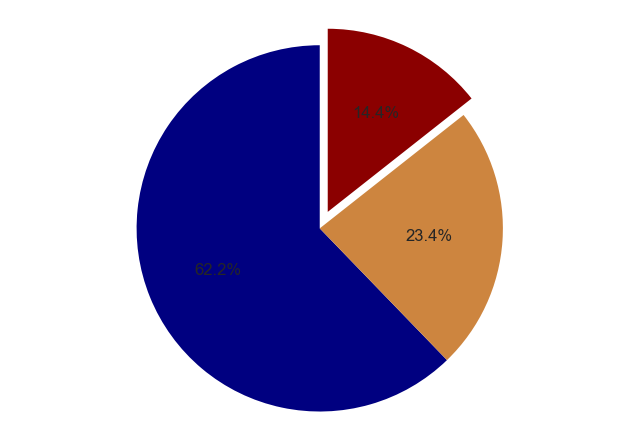## Share of Wealth held by Richest and Poorest¶

In :
#Filter
share=df.query("VAR in['ST1','ST10','ST5','SB60']" )

#Sort them and keep latest
share=share.sort_values(['Country', 'Time', 'VAR'], ascending=False)

#Reshape it
share=share.pivot(index='Country', columns='Variable', values='tranformed_value')

#Create average
share.loc['OECD 28 Average']=share.mean()
share

Out:
Variable Share of bottom 60% of wealth Share of top 1% of wealth Share of top 10% of wealth Share of top 5% of wealth
Country
Australia 16.535755 14.997084 46.465977 33.513176
Austria 8.004402 25.527975 55.590530 43.521667
Belgium 18.985046 12.056460 42.495087 29.708549
Canada 12.485457 15.502501 50.309025 36.498627
Chile 8.490582 17.398533 57.710564 42.665962
Denmark -3.851432 23.620983 63.976688 47.340378
Estonia 12.786300 21.227100 55.713390 43.189041
Finland 13.552249 13.307406 45.231823 31.374777
France 12.110005 18.645706 50.586899 37.260921
Germany 6.456923 23.661791 59.758945 46.255795
Greece 17.925282 9.155185 42.423824 28.775896
Hungary 15.445245 17.226322 48.477074 35.642178
Ireland 7.184053 14.179169 53.792400 37.657055
Italy 17.315496 11.686905 42.784328 29.652973
Japan 17.749653 10.766300 41.016430 27.691130
Korea 17.694309 NaN NaN NaN
Latvia 7.114193 21.386295 63.376293 49.108349
Luxembourg 15.277357 18.805170 48.667435 36.296368
Netherlands -3.985713 27.828711 68.347847 52.458202
New Zealand 12.318701 NaN 52.944607 39.701721
Norway 7.308864 20.128822 51.450287 37.845356
Poland 18.263699 11.729097 41.839970 29.011663
Portugal 12.422934 14.442963 52.127502 36.539959
Slovak Republic 25.886946 9.317052 34.325706 22.962288
Slovenia 17.293293 23.032787 48.622921 37.912071
Spain 18.664709 16.324175 45.577541 33.258755
United Kingdom 12.065424 20.452438 52.497028 38.812523
United States 2.398355 42.476238 79.471603 68.048569
OECD 28 Average 12.282075 18.264737 51.688212 38.248294

### Graph 4- Share of wealth held by richest and poorest¶

In :
##Clear
plt.clf()

# Set up the matplotlib figure
f, ax = plt.subplots(figsize=(11, 20))

#Use seaborn's heatmap function

#Set the order of columns
order=['Share of top 1% of wealth'
,'Share of top 5% of wealth'
,'Share of top 10% of wealth'
,'Share of bottom 60% of wealth']

sns.heatmap(share[order], cmap="YlGnBu", vmin=0,vmax=80, center=50,
square=True, linewidths=.5, cbar_kws={"shrink": .5}, annot=True)

ax.xaxis.tick_top()
y_pos = np.arange(len(share.columns))

plt.xticks(y_pos+1.8, order,  fontsize = 12,rotation=40,)
plt.ylabel('')
plt.xlabel('')

plt.savefig(root+'f4.png')
plt.show()

<matplotlib.figure.Figure at 0xcd1c940>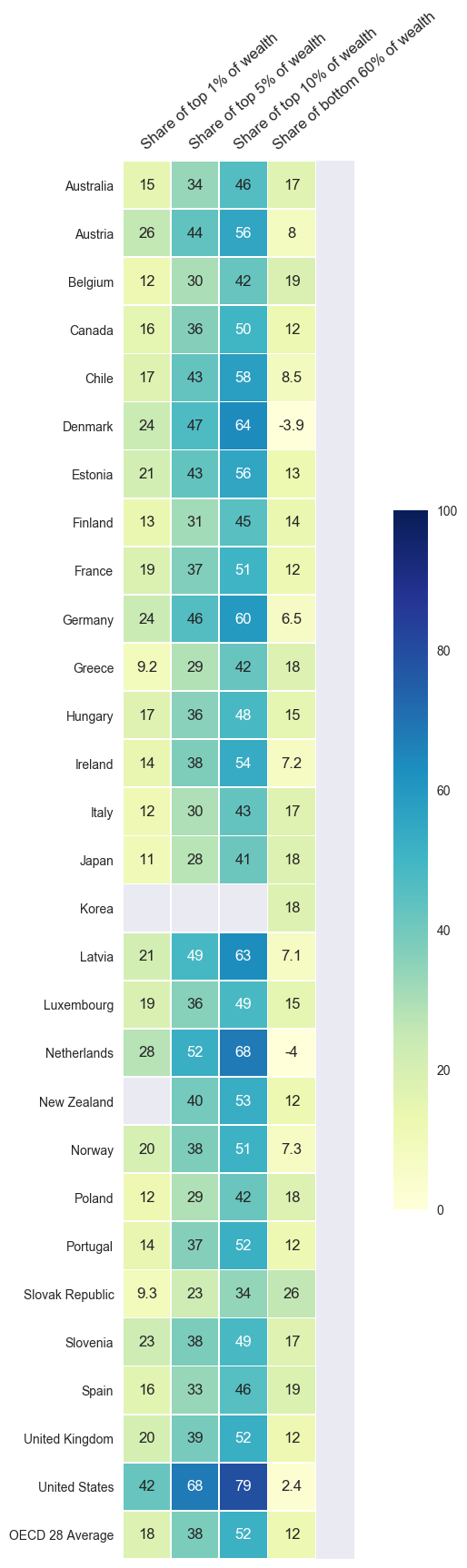## Debt¶

In :
#Filter debt variables

debt=df.query("VAR in['T4C5', 'T4C4','PIHR3','PIH']" )

#Select latest year
s_debt=debt.sort_values(['Country', 'Time'], ascending=False)
s_debtf=s_debt.pivot(index='Country', columns='Variable', values='tranformed_value')

#Shorten the name of some variables
s_debtf=s_debtf.reset_index()
s_debtf=s_debtf.rename(columns={'Share of indebted households with debt-to-income ratio above 3':'pct_debt_ratio_3'})
s_debtf=s_debtf.rename(columns={'Share of indebted households':'debt'})
s_debtf=s_debtf.rename(columns={'Median debt-to-income ratio of indebted households':'median_debt_income'})


Out:
Variable Country Median debt-to-assets ratio of indebted households median_debt_income debt pct_debt_ratio_3
0 Australia 22.340000 91.010002 71.765266 18.992840
1 Austria 20.100000 32.700001 34.413723 3.301259
2 Belgium 18.700001 79.799995 48.386173 6.875115
3 Canada 44.000000 161.000000 45.395592 11.759620
4 Chile 10.481910 14.145970 71.906647 29.658066

### Graph Debt¶

In :
#Graph header
plt.clf()
fig=plt.figure(figsize=(15, 10))

#We will graph three variables. The size of the circle will be used to denote the variable  pct_debt_ratio_3
s_debtf['color']=''
s_debtf.loc[(s_debtf['pct_debt_ratio_3']>0) & (s_debtf['pct_debt_ratio_3']<=10), 'color']='navy'
s_debtf.loc[(s_debtf['pct_debt_ratio_3']>10) & (s_debtf['pct_debt_ratio_3']<20), 'color']='orange'
s_debtf.loc[(s_debtf['pct_debt_ratio_3']>=20) , 'color']='darkred'

#Place them on a list
color=s_debtf['color'].tolist()

#We place them on a list. This allows to modify individual location
locationx=(s_debtf['debt']).tolist()
locationy=(s_debtf['median_debt_income']).tolist()

#Change for NZ
locationy =locationy-2
#Change for NDL
locationy =locationy+2

#This parameter allows to modify the circle size.
size=s_debtf['pct_debt_ratio_3']*4000

#Plot graph
scat3=plt.scatter( s_debtf['debt'],
s_debtf['median_debt_income'],
marker='o',
label=s_debtf['Country'],
alpha=1,
c=s_debtf['pct_debt_ratio_3'],
cmap='brg',
s=size**(1/2.15))

plt.xlabel('Share of indebted households')
plt.ylabel('Median debt-to-income ratio of indebted households')
plt.title('')
plt.xticks(  fontsize = 9, ha="center")
plt.xlim([20,100])

change()
fmt = '%2.0f%%' # Format you want the ticks, e.g. '40%'
yticks = mtick.FormatStrFormatter(fmt)
ax.yaxis.set_major_formatter(yticks)
ax.xaxis.set_major_formatter(yticks)
m=plt.colorbar(scat3, ticks=[0,1, 10,20,30])
m.ax.set_title('Share of indebted households with debt-to-income ratio above 3')


<matplotlib.figure.Figure at 0xbd22630>